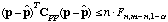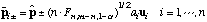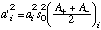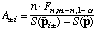# Manual Page for Command >>> LINEARITY

Syntax
>>> LINEARITY (: alpha (%))

Parent Command
>> ERROR

Subcommand
-

Description
The covariance matrix of the estimated parameters, Cpp, is calculated using linear error analysis:The confidence region around the estimated parameter set for the linearized case consists of those values p for whichwhere phat is the parameter vector at the optimum. The covariance matrix Cpp approximates the actual surface of the objective function at its minimum by a tangent hyperellipsoid under the assumption of normality and linearity. If the model is nonlinear, the coverage of the confidence region by the linear approximation may be very poor with respect to both its size and its shape. When using this command, it is assumed that the shape of the confidence region is close to ellipsoidal, and that the orientation of the hyperellipsoid in the n-dimensional parameter space is accurately obtained from the linear error analysis. Then, by only adjusting the size of the hyperellipsoid, we can better approximate the confidence region without losing the advantage of producing easily understandable results which are also simple to report. The procedure adopted here is based on a comparison of the actual objective function with the results from the linear approximation at discrete points in the parameter space. These test points p‘ are preferably located along the main axis of the hyperellipsoid, i.e.:Here, p+- are two test parameter sets on the i-th axis, the direction of which is given by the eigenvector ui of the covariance matrix Cpp. Note that the distance from the optimal parameter set p is selected as a multiple of the corresponding eigenvalue ai and the quantile of the F-distribution. This means that the correction is tailored to approximate the confidence region on a certain confidence level (1-alpha). The eigenvalues ai‘ which represent the length of the semiaxis are now corrected as follows:withFinally, the new covariance matrix is back-calculated from the eigenvectors ui and the updated eigenvalues ai‘. This correction procedure requires 2*n additional solutions of the direct problem and is thus relatively inexpensive. While the resulting confidence region is ellipsoidal by definition, the differences between S(p+i‘) and S(p-i‘) provide, as a byproduct of the correction procedure, some insight into the asymmetry of the true confidence region. The user may specify alpha or (1-alpha) in % or the quantile n * Fn,m-n,1-alpha directly, (by omitting % on the command line). Note that the correction procedure fails if the minimum is not accurately identified. In this case iTOUGH2 automatically proceeds with the covariance matrix from the linear error analysis.

Example
> COMPUTATION
>> ERROR analysis
>>> calculate finite difference HESSIAN and then
>>> check LINEARITY assumption on the : 95 % confidence level
<<<
<<